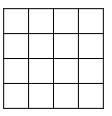Calculations from geometry:
The ratios of the sides of the quadrilateral are 3 : 6:4.5 : 3.5. Calculate their lengths if the circumference is 51 cm.

The sizes of the angles in the quadrilateral are equal to 29°30', 133°10', and 165°20'. What is the size of angle D?

a =  9 cm
b =  18 cm
c =  13.5 cm
d =  10.5 cm
D =  32 °

### Step-by-step explanation:Did you find an error or inaccuracy? Feel free to write us. Thank you!

Tips for related online calculators
Need help calculating sum, simplifying, or multiplying fractions? Try our fraction calculator.
Check out our ratio calculator.

#### Grade of the word problem:

We encourage you to watch this tutorial video on this math problem: